Reach Us+441474556909
Modeling and Control of a Wind System Based Doubly Fed Induction Generator: Optimization of the Power Produced | OMICS International
Journal of Electrical & Electronic Systems
All submissions of the EM system will be redirected to Online Manuscript Submission System. Authors are requested to submit articles directly to Online Manuscript Submission System of respective journal.

# Modeling and Control of a Wind System Based Doubly Fed Induction Generator: Optimization of the Power Produced

Marouan Elazzaoui*

Department of Electrical Engineering, Electronic Power and Control Laboratory, Mohammedia School of Engineering Université Mohammed V-Agdal, Morocco

*Corresponding Author:
Marouan Elazzaoui
Department of Electrical Engineering
Electronic Power and Control Laboratory
Mohammedia School of Engineering Université Mohammed V-Agdal, Morocco
E-mail: [email protected]

Received Date: January 15, 2015 Accepted Date: March 03, 2015 Published Date: April 04, 2015

Citation: Elazzaoui M (2015) Modeling and Control of a Wind System Based Doubly Fed Induction Generator: Optimization of the Power Produced. J Electr Electron Syst 4:141. doi:10.4172/2332-0796.1000141

Copyright: © 2015 Elazzaoui M. This is an open-access article distributed under the terms of the Creative Commons Attribution License, which permits unrestricted use, distribution, and reproduction in any medium, provided the original author and source are credited.

Visit for more related articles at Journal of Electrical & Electronic Systems

#### Abstract

This paper deals with the modeling and control of a wind energy system based on a doubly-fed induction generator. Initially, an MPPT control strategy of the doubly-fed induction generator is presented. Thereafter, the control vectororiented stator flux is performed. Finally, the simulation results of the wind system using a doubly-fed of 3 MW are presented in the Matlab / Simulink environment.

#### Keywords

Wind turbine; Doubly-fed induction generator; Converter; Vector control

#### Introduction

Today, wind energy has become a viable solution for energy production, in addition to other renewable energy sources. While the majority of wind turbines are fixed speed, the number of variable speed wind turbines is increasing . The doubly-fed induction generator with vector control is a machine that has excellent performance and is commonly used in the wind turbine industry . There are many reasons for using a doubly-fed induction generator for a variable speed wind turbine; such as reducing efforts on the mechanical parts, reducing noise and the possibility of control of active and reactive power . The wind system using DFIG and a “back-to-back” converter that connects the rotor of the generator and the network has many advantages (Figure 1).

One advantage of this structure is that the power converters used are dimensioned to pass a fraction of the total power of the system [4,5]. Thereby reducing losses in power electronic components. The performance and power production does not only depend on the DFIG, but also the way in which the two parts of “back-to-back” converter is controlled. The power converter machine side is called «Rotor Side Converter» (RSC) and the grid side power converter is called «Grid Side Converter» (GSC). The machine side power converter controls the active power and reactive power produced by the machine. As for the grid-side converter, it controls the DC bus voltage and line-side power factor. In this paper, we present a technique for controlling the two power converters. We will analyze their dynamic performance by simulations in Matlab / Simulink environment. We start with a model of the wind turbine,then a technique for continued operation at maximum power point tracking (MPPT) will be presented. Subsequently, we present a model of DFIG in the landmark Park, and the general principle of the control of two power converters. We conclude by presenting the simulation results and their interpretation.

#### Modeling of Wind Turbine

By applying the theory of momentum and Bernoulli’s theorem, we can determine the incident power (the theoretical power) due to wind [6,7]: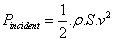(1)

S : the surface swept by the blades of the turbine m2

ρ : the density of air (ρ =1,225kg / m2 at atmospheric pressure).

v : wind speed (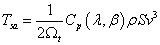m/s)

In a wind energy system, due to various losses, the power extracted from provided on the rotor of the turbine is less than the incident power. The power extracted is expressed by :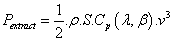(2)

Cp (λ,β) is called the power coefficient, which expresses the aerodynamic efficiency of the turbine. It depends on the ratio, which is the ratio between the speed at the end of the blades and the wind speed, and the orientation angle β of the blades. The ratio λ can be expressed by the following equation (8,9):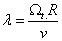(3)

Ω: The turbine speed of rotation (rad/s).

R: The length of a blade.

The maximum of power coefficient Cp was determined by Albert Betz as follows: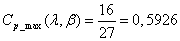(4)

The power coefficient is intrinsic to the formation of wind turbine and depends on the profiles of the blades. The power coefficient can be modeled with a single equation that depends on the speed ratio λ and the orientation angle β of the blades as follows: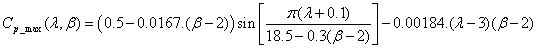(5)

The Figure 2 shows curves of the power coefficient as a function of λ for various β values. This gives a maximum power coefficient of 0.5 for a speed ratio λ which is 9.13 maintaining β at 2°. By setting β and λ respectively to their optimal values, the wind system will provide optimum electrical power.

The aerodynamic torque on the slow axis is expressed by:(6)

Mechanical speed is related to the speed of rotation of the turbine by the coefficient of the gearbox. The torque on the slow axis is connected to the torque on the fast axis (generator side) by the coefficient of the gearbox (Figure 3).

The total inertia J is made up of the inertia of the turbine plotted on the generator rotor and inertia of the generator: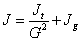(7)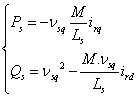Jt : inertia of the turbine.

Jg : inertia of the generator.

To determine the evolution of the mechanical speed from total torque Tmec applied to the rotor of DFIG, we apply the fundamental equation of dynamics: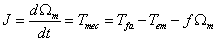(8)

Ω: mechanical speed of DFIG.

Tfa : aerodynamic torque on the fast axis of the turbine.

Tem: Electromagnetic torque.

m : coefficient of friction.The previous equations used to establish the block diagram of the turbine model.

#### Maximum Power Extraction

In order to capture the maximum power of the incident wind, permanently must adjust the rotational speed of the turbine to the wind. An erroneous speed measurement therefore inevitably leads to degradation of the received power that is why most wind turbines are controlled without control of the speed. The controller in this case should impose a reference torque to allow DFIG turning at an adjustable speed to ensure optimal operating point of power extraction. In this context, the ratio of the speed of wind λ must be maintained at its optimum value λ=λopt on a certain wind speed range. Thus, the power coefficient would be maintained at its maximum value (Figure 4).

#### Modelling of DFIG

The electrical equations of DFIG in the dq reference can be written equation 9,11: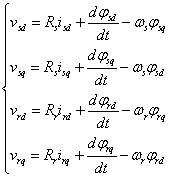(9)

The pulse of the stator currents being constant, the rotor pulse is derived by: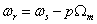(10)

The equations for the flux in dq reference are given by: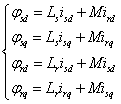With: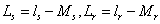:Cyclic inductances of stator and rotor phase. ls et lr inductors own stator and rotor of the machine. Ms et Mr mutual inductances between two stator phases and between two rotor phases of the machine.

M: maximum mutual inductance between stator and rotor stage.

p: number of pairs of poles of the DFIG.The expression of the electromagnetic torque of the DFIG based on the flow and stator currents is written as follows: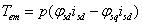(12)

The active and reactive power of the stator and rotor are written as follows (9,11):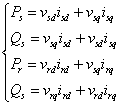(13)

#### Vector Control of DFIG

DFIG control strategies are based on two different approaches (12):

• Flow control in closed loop, where the frequency and voltage are considered variables (unstable grid).

• Flow control in open loop when the voltage and frequency are constant (stable grid).

In our study, the frequency and voltage are assumed to be constant. We can see from equation (12), the strong coupling between flows and currents. Indeed, the electromagnetic torque is the cross product between flows and stator currents, making the control of DFIG particularly difficult. To simplify ordering, we approximate the model to that of the DC machine which has the advantage of having a natural coupling between flows and currents. For this, we apply vector control, also known order by direction of flow. We choose dq reference linked to the rotating field (Figure 5).

Stator flux φs is oriented along the axis. Thus, we can write: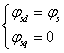(14)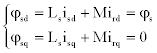(15)

In the field of production of wind energy, these are average machines and high power which are mainly used. Thus, we neglect the stator resistance. Taking the constant stator flux we can write: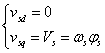(16)

To determine the angles necessary for transformation Park of the stator variables θs and the rotor variables θr, we used a phase locked loop (PLL) as shown in Figure 6. This PLL allows to accurately estimate the frequency and amplitude of the grid (13). The architecture of the controller is shown in Figure 7. It is based on the three-phase model of the electromechanical conversion chain of the wind energy system. From Figure 7, three commands are needed:

• The maximum extraction control wind power by controlling said MPPT (detailed in Section III).

• Control of RSC by controlling the electromagnetic torque and stator reactive power of DFIG.

• Control of GSC by controlling the voltage of the DC bus and the active and reactive power exchanged with the grid.

Control of the rotor side converter (RSC)

The principle of the Control of the rotor side converter is shown in Figure 8. The Controls of electromagnetic torque and stator reactive power will be obtained by controlling the rotor dq axes currents of DFIG. From the equations (15) and (16) we obtain the expression of the stator current: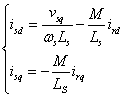(17)

These expressions are then substituted in the equation (11) of the rotor flux which then become: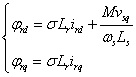(18)

With: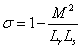:the dispersion coefficient of the DFIG Substituting the expressions of the direct and quadrature components of the rotor flux in the equation (9) we get: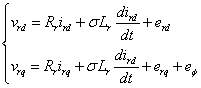With: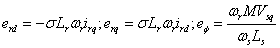The expression of the electromagnetic torque becomes: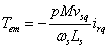(20)

Stator active and reactive powers are expressed by: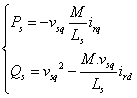(21)

These last expressions show that the choice of coordinate system (dq) makes the electromagnetic torque produced by the DFIG, and therefore the stator power, proportional to the current of the rotor axis (q). The stator reactive power, in turn, is proportional to the current of the rotor axis (d) due to a constant imposed by the grid. Thus, these stator powers can be controlled independently of one another. This shows us that we can set up a control rotor currents due to the influence of the couplings, every current can be controlled independently each with its own controller. The reference values for these regulators will be the rotor axis current (q) and the rotor axis current (d). The block diagram of the control loops of the axis rotor currents (dq) is shown in Figure 9, the regulators used are PI correctors.

The rotor current of the reference axis (q) is derived from the MPPT control via the electromagnetic torque reference (Figure 4). The rotor current of the reference axis (d) is, in turn, derived from the control of the stator reactive power.

From the equations (20) and (21) we obtain: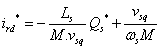(22)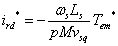(23)

Figure 10 shows the block diagram of the control of RSC. Figure 11 show the principle of the Control of the rotor side converter performs the following two functions:

• Control currents flowing in the RL filter.

• Control voltage of the DC bus.

Control currents flowing in the RL filter

According to Kirchhoff’s laws, the equations of the filter in the three-phase reference voltages are given by: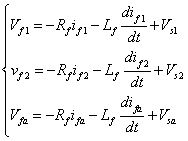(24)

Applying the Park transformation, we obtain: Pf*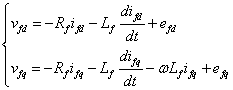(25)

With: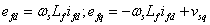The pattern of binding of GSC to grid in the landmark Park along the stator rotating field shows us that we can put in place a control of the currents flowing in the RL filter is given to the influence of the couplings, every axis can be controlled independently with each its own PI controller. The reference values for these controllers will be current in RL filter axes (dq) (Figure 12). The reference currents ifd * and ifd * are respectively the voltage from the control block of the DC bus and control of reactive power at the GSC connection point to the grid (Figure 11).

Neglecting losses in the resistance of RL filter and taking the orientation of the coordinate system (dq) connected to the rotary stator field vsd=0 the equations for the powers generated by the GSC are given by: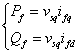(26)

From these equations, it is possible to impose the active and reactive power reference noted here Pf * and Qf * imposing the following reference currents: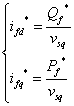(27)

Control of the DC bus voltage

We can express the powers involved on the DC bus by: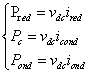(28)

These powers are linked by the relation: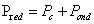(29)

Neglecting all the Joule losses (losses in the capacitor, the converter and the RL filter), we can write: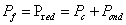(30)

By adjusting the power Pf, then it is possible to control the power Pc in the capacitor and therefore to regulate the DC bus voltage. To do this, the Pond and powers must be known to determine Pf *. The reference power for the capacitor is connected to the reference current flowing through the capacitor: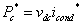(31)

Regulating the DC bus voltage is then effected by an external loop (with respect to the inner loop control of current), for maintaining a constant voltage on the DC bus, with a PI controller that generates the reference current ic * in the capacitor (Figure 13). Figure 14 shows the block diagram of the control of GSC. This block diagram includes the terms of decoupling and compensation to be able to independently control the (dq) axes currents circulating in the RL filter and the active and reactive power exchanged between the GSC and the grid.

#### Simulation Results

The simulations of the whole system were performed with Matlab/ Simulink, the DC bus reference voltage, denoted Vdc * is set at 1200 V. The reactive power references Qs * and Qf * are set to 0 VAr, ensuring unity power factor. We present in this section the results of the proposed control. Figure 15 illustrates the profile of the average wind speed used for simulation, while Figure 16 shows the shaft rotational speed derived by the turbine. The figures (Figures 17 and 18) show that the electromagnetic torque and reactive power provided by DFIG follow their references, this is due to control of direct and quadrature components of the rotor current. Figure 19 illustrates the power stator extracted, and Figure 20 shows that the DC bus voltage is perfectly regulated. We see in Figure 21 that the current delivered by the wind system is in phase with respect to the supply voltage, this confirms that the wind system injects the active power into the grid.

#### Conclusion

This paper is devoted to modeling, simulation and analysis of a wind turbine operating at variable speed. Stable operation of the wind energy system was obtained with the application of the control direction of the flow. The overall operation of the wind turbine and its control system were illustrated in transient and permanent regimes. DFIG operates in two quadrants. Operation hyposynchronous for positive slip and hypersynchronous operation for a negative slippage. The generator supplied power to the grid with an active power regardless of the mode of operation. The control strategy based on the PI control with correction was tested. Simulation results show that the proposed wind energy system is feasible and has many benefits.

#### References

Select your language of interest to view the total content in your interested language

### Article Usage

• Total views: 14830
• [From(publication date):
June-2015 - Sep 18, 2019]
• Breakdown by view type
• HTML page views : 10501

## Post your commentCan't read the image? click here to refresh
###### Peer Reviewed Journals

Make the best use of Scientific Research and information from our 700 + peer reviewed, Open Access Journals

International Conferences 2019-20

Meet Inspiring Speakers and Experts at our 3000+ Global Annual Meetings

Top